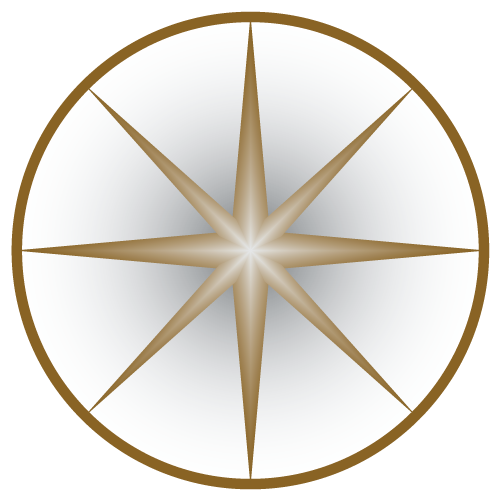INTERNATIONAL UNITS OF SI
7 BASE & 22 DERIVED UNITS
INTERNATIONAL UNITS OF SI
##### Conversion of SI to CGS Units Electric Charge, q 1 C (SI) = (10−1 c) Fr (CGS) Electric Current, I 1 A (SI) = (10−1 c) Fr/s (CGS) Electric Potential, φ · V 1 V (SI) = (108 csup>−1) statV (CGS) Electric Field, E 1 V/m (SI) = (106 c−1) statV/cm (CGS) Magnetic Induction, B 1 T (SI) = (104) Gs (CGS) Magnetic Field Strength, H 1 A/m (SI) = (4π 10−3) Oe (CGS) Magnetic Dipole Moment, μ 1 A·m² (SI) = (103) erg/Gs (CGS) Magnetic Flux, Φm 1 Wb (SI) = (108) Gs·cm2 (CGS) Resistance, R 1 Ω (SI) = (109 c−2) s/cm (CGS) Resistivity, ρ 1 Ω·m (SI) = (1011 c−2) s (CGS) Capacitance, C 1 F (SI) = (10−9 c2) cm (CGS) Inductance, L 1 H (SI) = (109 c−2) s2/cm (CGS)